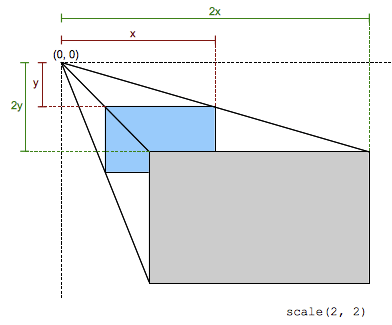/CSS

# scale()

The `scale()` CSS function defines a transformation that resizes an element on the 2D plane. Because the amount of scaling is defined by a vector, it can resize the horizontal and vertical dimensions at different scales. Its result is a `<transform-function>` data type.This scaling transformation is characterized by a two-dimensional vector. Its coordinates define how much scaling is done in each direction. If both coordinates are equal, the scaling is uniform (isotropic) and the aspect ratio of the element is preserved (this is a homothetic transformation).

When a coordinate value is outside the [-1, 1] range, the element grows along that dimension; when inside, it shrinks. If it is negative, the result a point reflection in that dimension. A value of 1 has no effect.

Note: The `scale()` function only scales in 2D. To scale in 3D, use `scale3d()` instead.

## Syntax

The `scale()` function is specified with either one or two values, which represent the amount of scaling to be applied in each direction.

```scale(sx)

scale(sx, sy)
```

### Values

`sx`
A `<number>` representing the abscissa of the scaling vector.
`sy`
A `<number>` representing the ordinate of the scaling vector. If not defined, its default value is `sx`, resulting in a uniform scaling that preserves the element's aspect ratio.
Cartesian coordinates on ℝ2 Homogeneous coordinates on ℝℙ2 Cartesian coordinates on ℝ3 Homogeneous coordinates on ℝℙ3
$\left(\begin{array}{cc}sx& 0\\ 0& sy\end{array}\right)$ $\left(\begin{array}{cc}sx0& 0\\ 0sy& 0\\ 0& 0& 1\end{array}\right)$ $\left(\begin{array}{cc}sx0& 0\\ 0sy& 0\\ 0& 0& 1\end{array}\right)$ $\left(\begin{array}{ccc}sx0& 0& 0\\ 0& sy& 0& 0\\ 0& 0& 1& 0\\ 0& 0& 0& 1\end{array}\right)$
`[sx 0 0 sy 0 0]`

## Examples

### Scaling the X and Y dimensions together

#### HTML

```<div>Normal</div>
<div class="scaled">Scaled</div>```

#### CSS

```div {
width: 80px;
height: 80px;
background-color: skyblue;
}

.scaled {
transform: scale(0.7); /* Equal to scaleX(0.7) scaleY(0.7) */
background-color: pink;
}
```

### Scaling X and Y dimensions separately, and translating the origin

#### HTML

```<div>Normal</div>
<div class="scaled">Scaled</div>```

#### CSS

```div {
width: 80px;
height: 80px;
background-color: skyblue;
}

.scaled {
transform: scale(2, 0.5); /* Equal to scaleX(2) scaleY(0.5) */
transform-origin: left;
background-color: pink;
}
```

## Browser compatibility

Please see the `<transform-function>` data type for compatibility info.